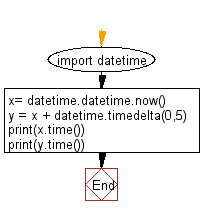﻿ Python: Add 5 seconds with the current time - w3resource# Python: Add 5 seconds with the current time

## Python datetime: Exercise-10 with Solution

Write a Python program to add 5 seconds with the current time.

Sample Solution:

Python Code:

``````import datetime
x= datetime.datetime.now()
y = x + datetime.timedelta(0,5)
print(x.time())
print(y.time())
```
```

Sample Output:

```12:37:43.350241
12:37:48.350241
```

Flowchart:## Visualize Python code execution:

The following tool visualize what the computer is doing step-by-step as it executes the said program:

Python Code Editor:

What is the difficulty level of this exercise?

Test your Python skills with w3resource's quiz

﻿

## Python: Tips of the Day

Free up Memory:

• Manual garbage collection can be performed on timely or event based mechanism.
```import gc
collected_objects = gc.collect()
```